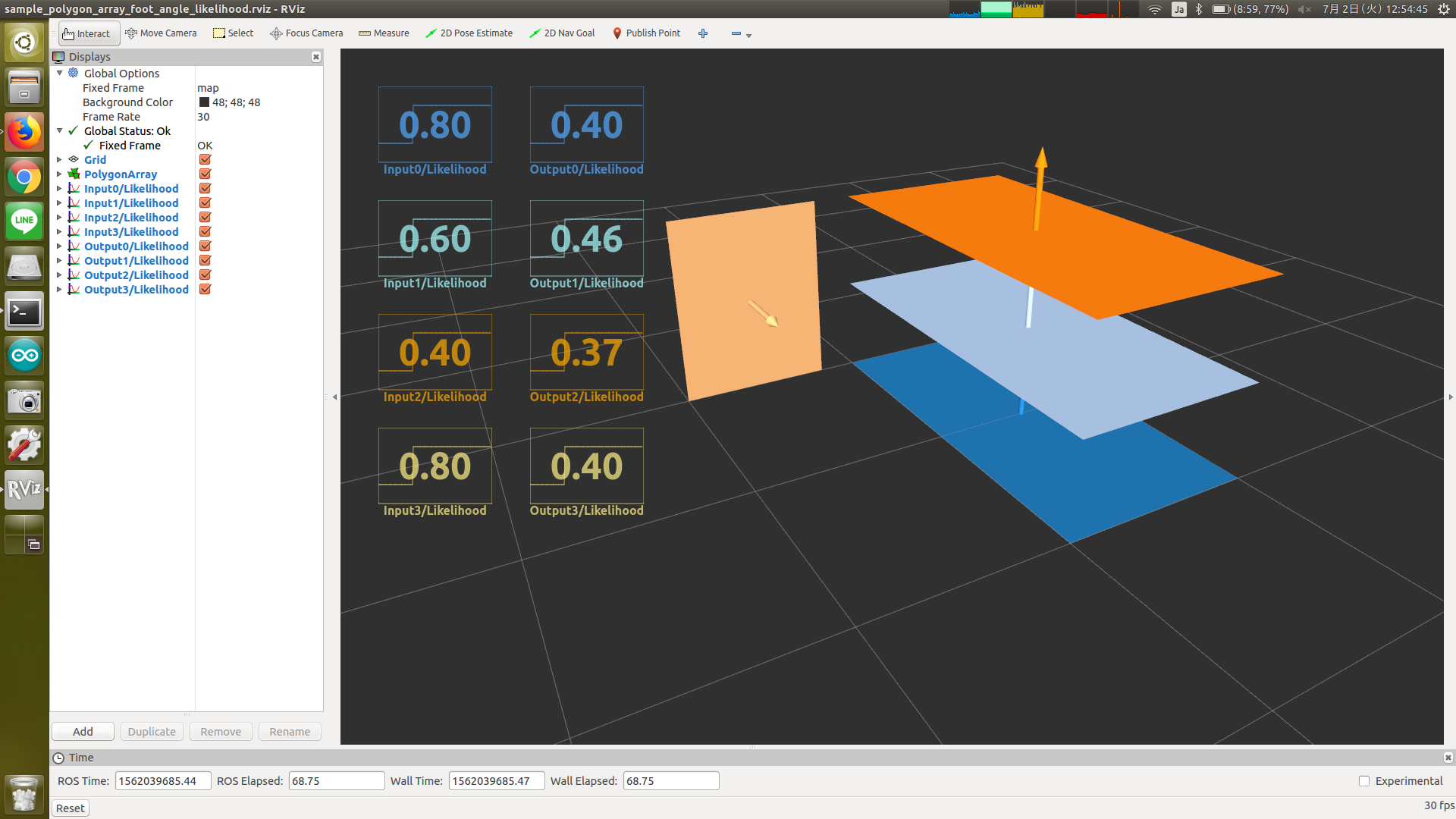# PolygonArrayFootAngleLikelihood¶Compute likelihood based on angular distance of foot from specified frame_id. The nearer polygon is, the larger likelihood is.

The likelihood is determined by 1/(1+d^2) where d is a angular difference from ~target_frame_id to the polygon.

## Subscribing Topic¶

• ~input (jsk_recognition_msgs/PolygonArray)

Input polygon array.

## Publishing Topic¶

• ~output (jsk_recognition_msgs/PolygonArray)

Output polygon array.

## Parameters¶

• ~target_frame_id (String, required)

Frame id to compute polygon’s distance from

• ~tf_queue_size (Int, default: 10)

Queue size of tf message filter

• ~axis (List of float, Default: [1, 0, 0])

Reference direction in ~target_frame_id coordinates system.

## Sample¶

roslaunch jsk_pcl_ros_utils sample_polygon_array_foot_angle_likelihood.launch